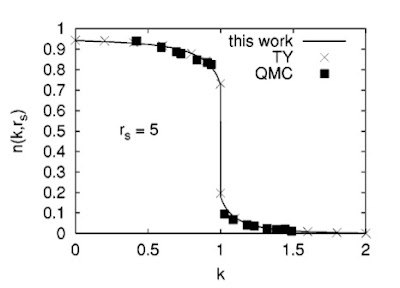## Friday, March 5, 2010

### Quantifying electronic correlations in simple metals

Considering natural orbitals in quantum chemistry this week reminded me of a key property of Fermi liquids: the existence of a discontinuity in the wavevector dependence of the Fourier transform of the one-electron reduced density matrix n(k).

The figure below is taken from a PRB paper by Paola Gori-Giorgi and Paul Ziesche. It shows the wavevector dependence of n(k) for a uniform electron liquid (at density corresponding to an interparticle spacing of rs=5, comparable to a typical metal). k=1 corresponds to the Fermi wavevector.For a non-interacting fermion system n(k)=1 for k less than 1 and 0 for k >1.

The figure below shows the magnitude of zF, the jump in n(k) at k=1, as a function of rs. Increasing rs corresponds to decreasing electron density and increasing correlation effects. This quantity zF can be related to the quasi-particle weight associated with the pole in the one-electron Greens function. The smaller zF the larger the effective mass of the quasi-particles.In a few weeks we will be looking at all this in the Quantum Many-Body Theory reading group.